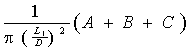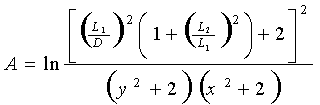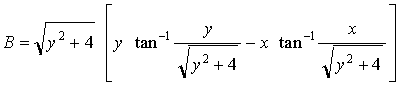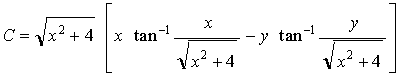Close

This page calculates the net radiant heat exchange for two isothermal (constant temperature) parallel square flat black plates of different edge lengths.The heat flow q from the plate is calculated as:

q = 56.69x10-9 x VF(1-2) x L12 x (T14 - T24)

Where 56.69x10-9 is the average Stefan-Bolzmann constant, L1 is the length of plate 1, T1 is the plate 1 temperature and T2 is the plate 2 temperature. The view factor VF(1-2) (also known as radiation shape factor, angle factor, and configuration factor) is defined as:

Length of plate 1 divided by the distance between the plates (L1 / D) must be greater than 0.2 for this calculation.

VF(1-2) =Where:Where X and Y are defined as:

X = (C) x ( 1 +E )
Y = (C) x ( 1 - E )
C = L1 / D
D = L2 / L1

Where L1 is the plate 1 length, L2 is the plate 2 length, and D is the distance between the plates.

References

Rosenow, W. M., J. P. Hartnett, Y. I. Young, Handbook of Heat Transfer, 3rd Ed., McGraw-Hill Handbooks, New York, 1998. p. 7.3 - 7.19, 7.81.